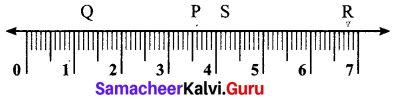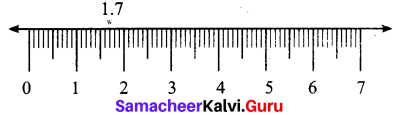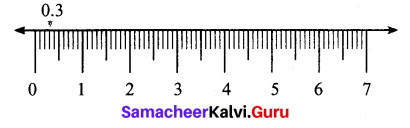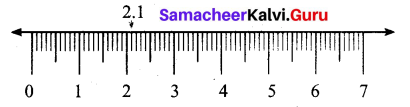Students can Download Maths Chapter 1 Number System Ex 1.4 Questions and Answers, Notes Pdf, Samacheer Kalvi 7th Maths Book Solutions Guide Pdf helps you to revise the complete Tamilnadu State Board New Syllabus and score more marks in your examinations.

## Tamilnadu Samacheer Kalvi 7th Maths Solutions Term 2 Chapter 1 Number System Ex 1.4

Question 1.
Write the decimal numbers represented by the points P, Q, R and S on the given number line.Solution:
The unit length between 1 and 2 is divided into 10 equal parts and the third part is taken as Q.
∴ Q represents 1 + 0.3 = 1.3
The unit lenth between 3 and 4 is divided into 10 equal parts and the 6th part is taken as P.
∴ P represents 3 + 0.6 = 3.6
The unit length between 4 and 5 is divied into 10 equal parts and the second part is taken as S.
∴ S represents 4 + 0.2 = 4.2
The unit length between 6 and 7 is divided into 10 equal parts and the 8th part is taken.
∴ R represents 6 + 0.8 = 6.8
P(3.6), Q(1.3), R(6.8), S(4.2).Question 2.
Represent the following decimal numbers on the number line.
(i) 1.7
(ii) 0.3
(iii) 2.1
Solution:
(i) 1.7
We know that 1.7 is more than 1, but less than 2.
There are one ones and 7 tenths in it. Divide the unit length between 1 and 2 on the number line into 10 equal parts and take 7 parts which represents 1.7 = 1 + 0.7(ii) 0.3
We know that 0.3 is more than 0, but less than 1.
There are 3 tenths in it. Divide the unit lenght between 0 and 1 on the number line into 10 equal parts and take 3 parts, which represent 0.3.(iii) 2.1
We knowthat 2.1 is more than 2 and less than 3.
There are 2 ones and 1 tenths in it.
Divide the unit length between 2 and 3 into 10 equal parts and take 1 part, which represemt 2.1 = 2 + 0.1Question 3.
Between which two whole numbers, the following decimal numbers lie?
(i) 3.3
(ii) 2.5
(iii) 0.9
Solution:
(i) 3.3 – 3.3 lies between 3 and 4.
(ii) 2.5 – 2.5 lies between 2 and 3.
(iii) 0.9 – 0.9 lies between 0 and 1.

Question 4.
Find the greater decimal number in the following.
(i) 2.3,3.2
(ii) 5.6,6.5
(iii) 1.2,2.1
Solution:
(i) 2.3, 3.2
Comparing the whole number parts of 2.3 and 3.2 we get 3 > 2.
3.2 > 2.3 – Greater number is 3.2

(ii) 5.6, 6.5
Comparing the whole number parts of 5.6 and 6.5, we get 6 > 5.
6.5 > 5.6 – Greater number is 6.5

(iii) 1.2, 2.1
Comparing the whole number parts of 1.2 and 2.1, we get 2 > 1.
2.1 > 1.2 – Greater number is 2.1Question 5.
Find the smaller decimal number in the following.
(i) 25.3,25.03
(ii) 7.01,7.3
(iii) 5.6,6.05
Solution:
(i) 25.3, 25.03
The whole number parts of both the numbers are equal.
∴ Comparing the digits at tenths place we get 0 < 3.
∴ 25.03 < 25.3 – Smaller number 25.03

(ii) 7.01,7.3
The whole number parts of both the numbers are equal.
Comparing the digits at tenths place we get 0 < 3.
∴ 7.01 < 7.3 – Smaller number is 7.01.

(iii) 5.6, 6.05
Comparing the whole number parts, we get 5 < 6.
∴ 5.6 < 6.05 – Smaller number is 5.6Objective Question

Question 6.
Between which two whole numbers 1.7 lie?
(i) 2 and 3
(ii) 3 and 4
(iii) 1 and 2